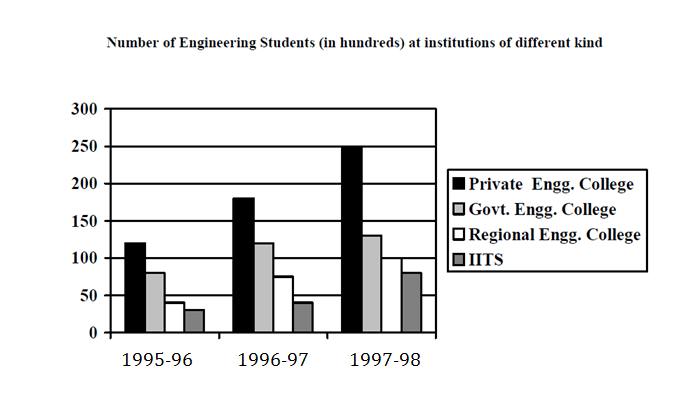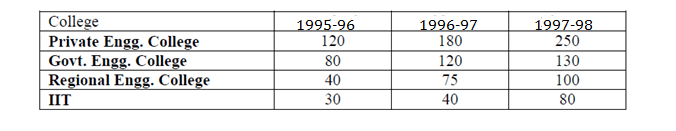Direction for 1 to 4: Analyze the table and answer the questions carefullyQuestion 1. The growth rate in students of Govt. Engg. Colleges compared to that of Private Engg. Colleges between 1995 – 96 and 1996 – 97 is
(a) more
(b) less
(c) equal
(d) 3/2

Question 2. What was the total number of engineering students in 1996 – 97?
(a) 28500
(b) 4400
(c) 4200
(d) 42000

Question 3. In 1997 – 98, what percent of Engg. Students were studying at IIT’s?
(a) 16
(b) 15
(c) 14
(d) 12

Question 4. The total number of Engg. Students in 1998 – 99, assuming a 10% reduction in the number over the previous year, is
(a) 5700
(b) 57000
(c) 44800
(d) none of these

AnalysisGrowth rate (Govt. Engg. College) = (120 – 80) / 80 = 50%

Growth rate (Private Engg. College) = (180 – 120) / 120 = 50%.

Hence the growth rate is equal.

From the table we can say that Total number of students in 1996–97 = (180 + 120 + 75 + 40) x 100 = 41500 = 42000 (approx)